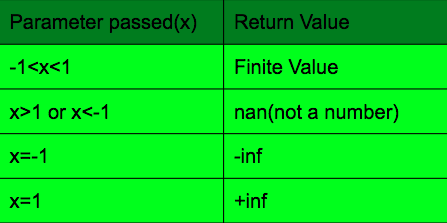# atanh() function in C++ STL

The atanh() is an inbuilt function in C++ STL which returns the inverse hyperbolic tangent of an angle given in radians.

Syntax:

```atanh(data_type x)
```

Parameter: This function accepts one mandatory parameter x which specifies the inverse hyperbolic angle in radian which lies in the range [-1, 1]. The parameter can be of double, float or long double datatype.

Return Value: This function returns the inverse hyperbolic sine of the argument in radians depending on the parameter passed in the argument. The different return values are given in the table below:Below programs illustrate the above method:

Program 1:

 `// C++ program to illustrate ` `// the atanh() function ` `// all return values ` `#include ` `using` `namespace` `std; ` ` `  `int` `main() ` `{ ` `    ``// Return value when -11 ` `    ``x = -2; ` `    ``result = atanh(x); ` ` `  `    ``cout << ``"\natanh(-2) = "` `<< result << ``" radians\n"``; ` `    ``cout << ``"atanh(-2) = "` `<< result * 180 / 3.141592 ` `         ``<< ``" degrees\n"``; ` ` `  `    ``return` `0; ` `} `

Output:

```atanh(0) = 0 radians
atanh(0) = 0 degrees

atanh(-1) = -inf degrees

atanh(1) = inf degrees

atanh(-2) = -nan degrees
```

Errors and Exceptions: The function returns a no matching function error message when a string or any other data_type except the one which the function excepts is passed.

Program 2:

 `// C++ program to demonstrate ` `// the atanh() function  ` `// string passed ` `#include ` `using` `namespace` `std; ` ` `  `int` `main() ` `{ ` `    ``string x = ``"gfg"``; ` ` `  `    ``// Function call to calculate atanh(x) value ` `    ``double` `result = atanh(x); ` ` `  `    ``cout << ``"atanh(50.0) = "` `<< result << ``" radians"` `<< endl; ` `    ``cout << ``"atanh(50.0) = "` `<< result * 180 / 3.141592 ` `         ``<< ``" degrees"` `<< endl; ` `    ``return` `0; ` `} `

Output:

```prog.cpp:11:25: error: no matching function for call to 'atanh(std::__cxx11::string&)'
double result = atanh(x);
```

Rated as one of the most sought after skills in the industry, own the basics of coding with our C++ STL Course and master the very concepts by intense problem-solving.

My Personal Notes arrow_drop_uppawanasipugmailcom

If you like GeeksforGeeks and would like to contribute, you can also write an article using contribute.geeksforgeeks.org or mail your article to contribute@geeksforgeeks.org. See your article appearing on the GeeksforGeeks main page and help other Geeks.

Please Improve this article if you find anything incorrect by clicking on the "Improve Article" button below.

Improved By : AlexTs

Article Tags :
Practice Tags :

Be the First to upvote.

Please write to us at contribute@geeksforgeeks.org to report any issue with the above content.